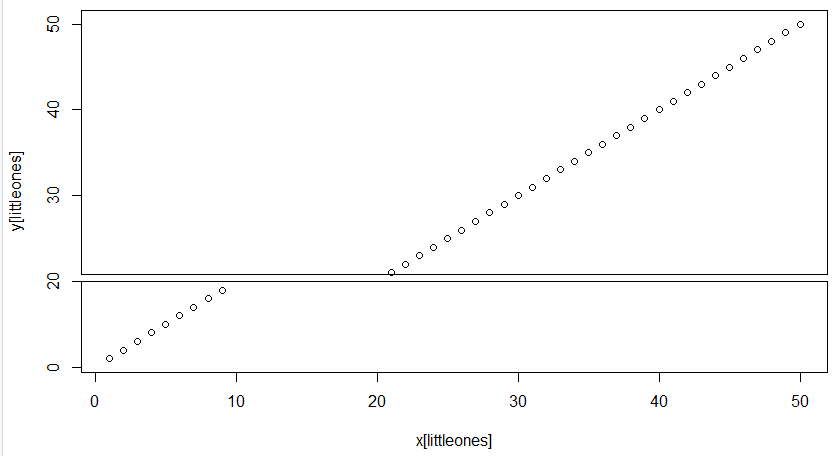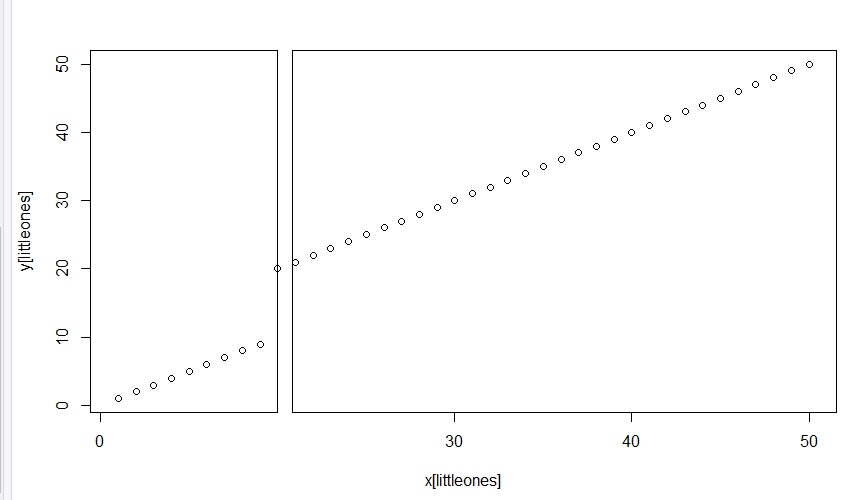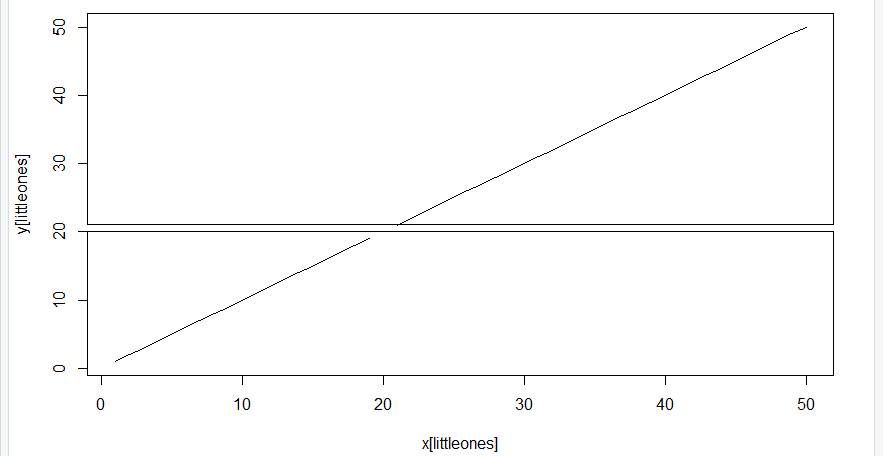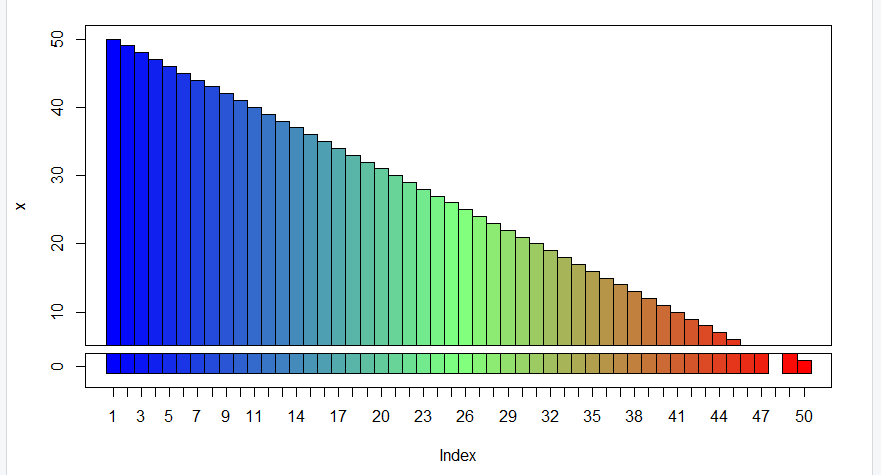GFG App
Open AppBrowser
Continue

In this article, we will discuss the break axis of the plot with its working examples in the R programming language

## Methodn1: Break Y-Axis of Plot Using gap.plot() Function of plotrix Package

In this method break y-axis of the plot using the gap.plot() Function of plotrix Package, the user first needs to install and import the plotrix package to the working console of the R, further the user needs to call the gap.plot() function further pass the data created for the plot and the gap coordinates as per requirements of the user as the parameters to get the break y-axis of plot accordingly in the R programming language.

Syntax to install and import the plotrix package in the R console:

```install.packages("plotrix")
library("plotrix")```

gap.plot() function: This function displays a plot with one or two missing ranges on one of the axes.

Syntax: gap.plot(x,y,gap)

Arguments::

• x,y: data values
• gap: the range(s) of values to be left out

Example:

In this example, we have created the Break Y-Axis of Plot at the 10, 20 coordinates of the scatter plot created of the data 50 to 1 in both the axis using the gap.plot() function in the R programming language.

## R

 `# Import required libraries  ` `library``(``"plotrix"``) ` ` `  `# Create data ` `x<-50:1 ` `y<-50:1 ` ` `  `# Apply gap.plot function ` `gap.plot``(x, y,gap = ``c``(10, 20)) `

Output:## Method 2: Break X-Axis of Plot Using gap.plot() Function of plotrix Package

In this method break x-axis of the plot using the gap.plot() Function of plotrix Package, the user first needs to install and import the plotrix package to the working console of the R, further, the user needs to call the gap.plot() function further passes the data created for the plot and the gap coordinates as per requirements of the user and the here the user needs to the user the gap.axis set to x  as the parameters to get the break x-axis of plot accordingly in the R programming language.

Example:

In this example, we have created the Break X-Axis of Plot at the 10, 20 coordinates of the scatter plot created of the data 50 to 1 in both the axis using the gap.plot() function with the gap.,axis set to x-axis set as the parameter in the R programming language.

## R

 `# Import required libraries  ` `library``(``"plotrix"``) ` ` `  `# Create data ` `x<-50:1 ` `y<-50:1 ` ` `  `# Apply gap.plot function ` `gap.plot``(x, y,gap = ``c``(10, 20),gap.axis = ``"x"``) `

Output:## Method 3: Break Axis of the Line Plot Using gap.plot() Function of plotrix Package

In this method break y-axis of the plot using the gap.plot() Function of plotrix Package, the user needs to install and import the plotrix package to the working console of the R, further, the user needs to call the gap.plot() function further passes the data created for the plot and the gap coordinates as per requirements of the user with the special parameter needs since it is a line plot so the user needs to pass the type argument and set it to “l” to plot the line plot and add the break axis to it accordingly in the R programming language.

Example:

In this example, we have created the Break Y-Axis of Plot at the 20, 20 coordinates of the scatter plot created of the data 50 to 1 in both the axis using the gap.plot() function with the type set to “l” as the parameter in the R programming language.

## R

 `# Import required libraries  ` `library``(``"plotrix"``) ` ` `  `# Create data ` `x<-50:1 ` `y<-50:1 ` ` `  `# Apply gap.plot function ` `gap.plot``(x, y,gap = ``c``(20, 20),type=``"l"``) `

Output:## Method 4: Break Axis of the BarPlot Using gap.barplot() Function of plotrix Package

In this method break y-axis of the plot using the gap.barplot() Function of plotrix Package, the user needs to install and import the plotrix package to the working console of the R, further, the user needs to call the gap.barplot() function further passes the data created for the plot and the gap coordinates as per requirements of the user to add the break axis to it accordingly in the R programming language.

Example:

In this example, we have created the Break Y-Axis of Plot at the 2, 4 coordinates of the barplot created of the data 50 to 1 in both the axis using the gap.barplot() function in the R programming language.

## R

 `# Import required libraries  ` `library``(``"plotrix"``) ` ` `  `# Create data ` `x<-50:1 ` ` `  `# Apply gap.plot function ` `gap.barplot``(x,gap=``c``(2,4))`

Output:My Personal Notes arrow_drop_up